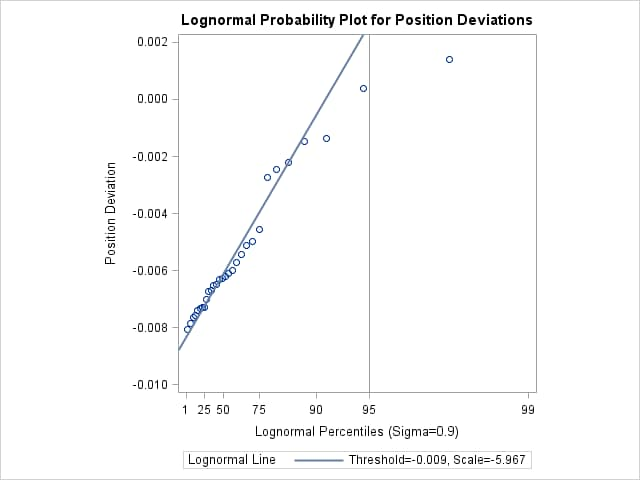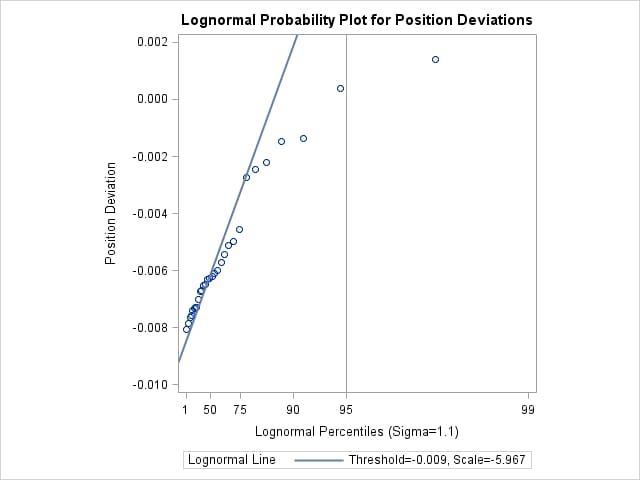# The UNIVARIATE Procedure

### Example 4.26 Creating Lognormal Probability Plots

This example is a continuation of the example explored in the section Modeling a Data Distribution.

In the normal probability plot shown in Figure 4.6, the nonlinearity of the point pattern indicates a departure from normality in the distribution of `Deviation`. Because the point pattern is curved with slope increasing from left to right, a theoretical distribution that is skewed to the right, such as a lognormal distribution, should provide a better fit than the normal distribution. See the section Interpretation of Quantile-Quantile and Probability Plots.

You can explore the possibility of a lognormal fit with a lognormal probability plot. When you request such a plot, you must specify the shape parameter for the lognormal distribution. This value must be positive, and typical values of range from 0.1 to 1.0. You can specify values for with the SIGMA= secondary option in the LOGNORMAL primary option, or you can specify that is to be estimated from the data.

The following statements illustrate the first approach by creating a series of three lognormal probability plots for the variable `Deviation` introduced in the section Modeling a Data Distribution:

```title 'Lognormal Probability Plot for Position Deviations';
ods graphics on;
proc univariate data=Aircraft noprint;
probplot Deviation /
lognormal(theta=est zeta=est sigma=0.7 0.9 1.1)
odstitle = title
href     = 95
square;
run;
```

The LOGNORMAL primary option requests plots based on the lognormal family of distributions, and the SIGMA= secondary option requests plots for equal to 0.7, 0.9, and 1.1. These plots are displayed in Output 4.26.1, Output 4.26.2, and Output 4.26.3, respectively. Alternatively, you can specify to be estimated using the sample standard deviation by using the option SIGMA=EST.

The SQUARE option displays the probability plot in a square format, the HREF= option requests a reference line at the 95th percentile.

Output 4.26.1: Probability Plot Based on Lognormal Distribution with =0.7Output 4.26.2: Probability Plot Based on Lognormal Distribution with =0.9Output 4.26.3: Probability Plot Based on Lognormal Distribution with =1.1The value in Output 4.26.2 most nearly linearizes the point pattern. The 95th percentile of the position deviation distribution seen in Output 4.26.2 is approximately 0.001, because this is the value corresponding to the intersection of the point pattern with the reference line.

Note: After the that produces the most linear fit is found, you can then estimate the threshold parameter and the scale parameter . See Example 4.31.

The following statements illustrate how you can create a lognormal probability plot for `Deviation` by using a local maximum likelihood estimate for .

```title 'Lognormal Probability Plot for Position Deviations';
proc univariate data=Aircraft noprint;
probplot Deviation / lognormal(theta=est zeta=est sigma=est)
href     = 95
odstitle = title
square;
run;
```

The plot is displayed in Output 4.26.4. Note that the maximum likelihood estimate of (in this case, 0.882) does not necessarily produce the most linear point pattern.

Output 4.26.4: Probability Plot Based on Lognormal Distribution with EstimatedA sample program for this example, uniex16.sas, is available in the SAS Sample Library for Base SAS software.